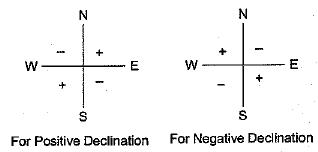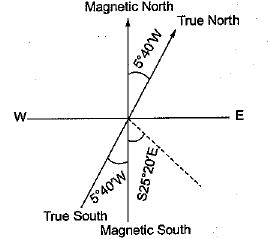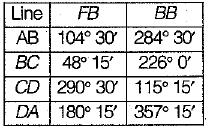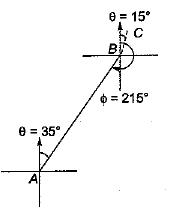Courses

# Compass Surveying And Theodolite - 2

## 10 Questions MCQ Test Topicwise Question Bank for GATE Civil Engineering | Compass Surveying And Theodolite - 2

Description
This mock test of Compass Surveying And Theodolite - 2 for Civil Engineering (CE) helps you for every Civil Engineering (CE) entrance exam. This contains 10 Multiple Choice Questions for Civil Engineering (CE) Compass Surveying And Theodolite - 2 (mcq) to study with solutions a complete question bank. The solved questions answers in this Compass Surveying And Theodolite - 2 quiz give you a good mix of easy questions and tough questions. Civil Engineering (CE) students definitely take this Compass Surveying And Theodolite - 2 exercise for a better result in the exam. You can find other Compass Surveying And Theodolite - 2 extra questions, long questions & short questions for Civil Engineering (CE) on EduRev as well by searching above.
QUESTION: 1

### If fore bearing of a line is S 49°52'E (assuming there is no local attraction), the back bearing of the line will be

Solution:

If the fore bearing of a line is given as the quadrantal bearing, then back bearing is numerically equal to the fore bearing. However N changes to S and Exchanges to W and vice versa.

QUESTION: 2

### If the declination is 5°40'W, which one of the following magnetic bearing would represent the true bearing of S 25°20'E?

Solution:

Note that negative (W) declination should be added to quadrantal bearing in second and fourth quadrant i.e. NθW or SθE and it should be subtracted from quadrantal bearing in first and third quadrant i.e., NθE and SθW. Reverse should be done for positive (E) declination.True bearing = S 25° 20'E + 5°40'
= S 31° 0'EQUESTION: 3

### The prismatic compass and surveyor’s compass

Solution:
QUESTION: 4

The following bearing were observed while traversing with a compassWhich stations were affected by local attraction?

Solution:

As the difference between F.B. and B.B. of line AB is exactly 180°, stations A and B are free from local attraction which is not the case with line CD.
Hence stations C and D are affected by local attraction.

QUESTION: 5

The multiplying constant of a theodolite is

Solution:
QUESTION: 6

Theodolite is an instrument used for

Solution:
QUESTION: 7

Agonic line is the line joining points having

Solution:
QUESTION: 8

Which one of the following is carried out by two theodolite method?

Solution:
QUESTION: 9

If the forebearing of a line AB is 35° and that of line BC 15° then the included angle between the lines is

Solution:BB of AB = FB + 180°
= 35° + 180° = 215°
∴ included angle between line,
AB and BC = 360° - 215° + 15° = 160°

QUESTION: 10

What is the magnetic declination at a place if the magnetic bearing of the sun at noon is 184°

Solution:

True bearing
= Magnetic bearing + Declination
∴ 180° = 184° + Declination
or Declination = -4° = 4°W